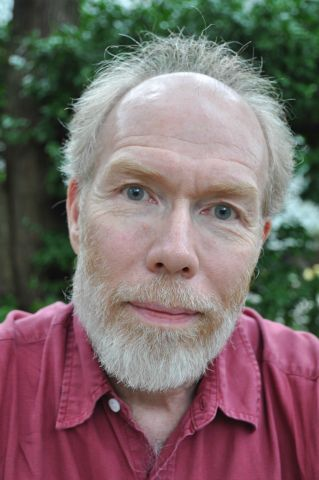Program for mathematics 2019

Visting Professor

Robert R. Bruner
Professor at Wayne State University, Detroit, USA

Nominated by:
KTH Royal Institute of Technology

Computer codes solve abstract mathematical problems

Robert R. Bruner is a professor at Wayne State University, Detroit, USA. Thanks to a grant from the Knut and Alice Wallenberg Foundation, he will be a visiting professor at the Department of Mathematics, KTH Royal Institute of Technology, Stockholm.

Visiting Professor Robert R. Bruner is an expert in the field of algebraic topology. Algebraic topology aims to describe geometric objects ("spaces") using algebraic methods. Unlike geometry, topology describes the aspects and properties of the space that are not affected by continuous deformations, the invariant properties.

Algebraic-topological methods have also become important in several other areas of mathematics, such as algebraic geometry or number theory. Of particular interest here is K-theory, a part of algebraic number theory that can be regarded as a topological invariant that is adapted so it can be used to study arithmetic issues.

Many results, methods and tools in algebraic topology are highly abstract. Even to actually calculate something that may seem simple to a mathematician, such as the deformation classes of transformations from a sphere of a higher dimension to a sphere of a lower dimension, knowledge of the theory is not enough. It also requires a lot of training in its practical application – knowledge that cannot be found in books or in articles.

Robert Bruner's focus has long been to make the theories, which he and other topologists developed, practically useful through the efficient performance of calculations on a computer. He is a world-leading researcher in the specific calculation of problems that arise within the K-theory. The main objective of the project is to studyK-theory’s central, but as yet unproven, conjecture, using appropriate computer codes and analytical methods.